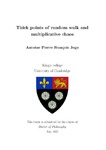## Thick points of random walk and multiplicative chaos

Jego, Antoine
##### DOIThesis
##### Abstract

This thesis concentrates on the classical problem of understanding the multifractal properties of Brownian motion and random walk. Specifically, we will be interested in the set of thick points, that is points where the trajectory goes back unusually often. The study of such points was initiated sixty years ago by Erdos and Taylor and has attracted a lot of attention, but we are able to make considerable progress on this topic by establishing a deep connection with an a priori unrelated area of Probability theory, called Gaussian multiplicative chaos.

Firstly, in two dimensions, we answer a question of Dembo, Peres, Rosen and Zeitouni and compute the number of thick points of planar random walk, assuming that the increments are symmetric and have a finite moment of order two. The proof provides a streamlined argument based on the connection to the Gaussian free field and works in a very general setting including isoradial graphs. In higher dimensions, we study the scaling limit of the set of thick points. In particular, we show that the rescaled number of thick points converges to a nondegenerate random variable and that the centred maximum of the local times converges to a randomly shifted Gumbel distribution.

Next, we construct the analogue of Gaussian multiplicative chaos measures for the local times of planar Brownian motion by exponentiating the square root of the local times of small circles. We also consider a flat measure supported on points whose local time is within a constant of the desired thickness level and show a simple relation between the two objects. Our results extend those of Bass, Burdzy and Khoshnevisan and in particular cover the entire L^1-phase or subcritical regime. These results allow us to obtain a nondegenerate limit for the appropriately rescaled size of thick points, thereby considerably refining estimates of Dembo, Peres, Rosen and Zeitouni.

Finally, we characterise the multiplicative chaos measure \Mc associated to planar Brownian motion by showing that it is the only random Borel measure satisfying a list of natural properties. These properties only serve to fix the average value of the measure and to express a spatial Markov property. As a consequence of our characterisation, we establish the scaling limit of the set of thick points of planar simple random walk, stopped at the first exit time of a domain, by showing the weak convergence towards \Mc of the point measure associated to the thick points. As a corollary, we obtain the convergence of the appropriately normalised number of thick points of random walk to a nondegenerate random variable.

2021-07-01